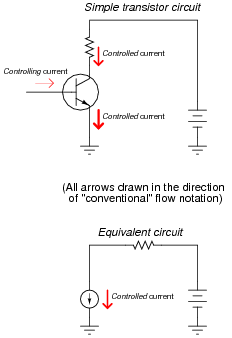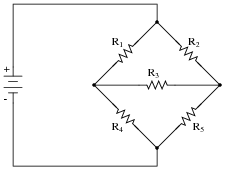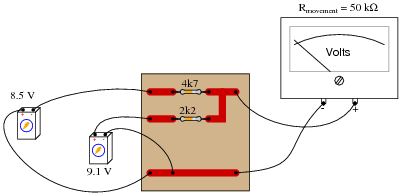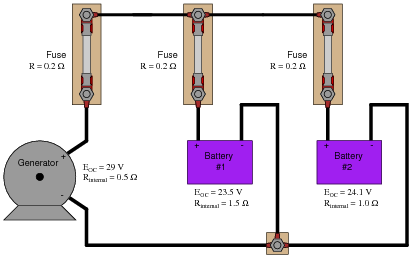# DC Branch Current Analysis

## Network Analysis Techniques

• #### Question 1

 Don’t just sit there! Build something!!

Learning to mathematically analyze circuits requires much study and practice. Typically, students practice by working through lots of sample problems and checking their answers against those provided by the textbook or the instructor. While this is good, there is a much better way.

You will learn much more by actually building and analyzing real circuits, letting your test equipment provide the “answers” instead of a book or another person. For successful circuit-building exercises, follow these steps:

1. Carefully measure and record all component values prior to circuit construction.
2. Draw the schematic diagram for the circuit to be analyzed.
3. Carefully build this circuit on a breadboard or other convenient medium.
4. Check the accuracy of the circuit’s construction, following each wire to each connection point, and verifying these elements one-by-one on the diagram.
5. Mathematically analyze the circuit, solving for all values of voltage, current, etc.
6. Carefully measure those quantities, to verify the accuracy of your analysis.
7. If there are any substantial errors (greater than a few percent), carefully check your circuit’s construction against the diagram, then carefully re-calculate the values and re-measure.

Avoid very high and very low resistor values, to avoid measurement errors caused by meter “loading”. I recommend resistors between 1 kΩ and 100 kΩ, unless, of course, the purpose of the circuit is to illustrate the effects of meter loading!

One way you can save time and reduce the possibility of error is to begin with a very simple circuit and incrementally add components to increase its complexity after each analysis, rather than building a whole new circuit for each practice problem. Another time-saving technique is to re-use the same components in a variety of different circuit configurations. This way, you won’t have to measure any component’s value more than once.

• #### Question 2

A transistor is a semiconductor device that acts as a constant-current regulator. For the sake of analysis, transistors are often considered as constant-current sources:Suppose we needed to calculate the amount of current drawn from the 6-volt source in this dual-source transistor circuit:We know the combined currents from the two voltage sources must add up to 5 mA, because Kirchhoff’s Current Law tells us that currents add algebraically at any node. Based on this knowledge, we may label the current through the 6-volt battery as “I”, and the current through the 7.2 volt battery as “5 mA − I”:Kirchhoff’s Voltage Law tells us that the algebraic sum of voltage drops around any “loop” in a circuit must equal zero. Based on all this data, calculate the value of I:Hint: simultaneous equations are not needed to solve this problem!

• #### Question 3

This transistor circuit is powered by two different voltage sources, one that outputs 6 volts, and the other that is variable.Transistors naturally act as current-regulating devices, and are often analyzed as though they were current sources. Suppose that this transistor happened to be regulating current at a value of 3.5 mA:How high does the voltage of the variable source have to be adjusted, until no current is drawn from the 6-volt battery?

Hint: simultaneous equations are not needed to solve this problem!

• #### Question 4

Describe, step-by-step, the steps required to calculate all currents and voltage drops in a DC network using the Branch Current Method.

• #### Question 5

While the “Branch Current” method may be used to analyze an unbalanced bridge circuit, it requires a lot of calculation! In this circuit, determine how many variables are needed to solve for all currents:• #### Question 6

Re-draw the circuit shown here into schematic form, and solve for the voltage drops across the two resistors using the “Branch Current” method:• #### Question 7

Calculate the amount of charging current through battery #1 using the Branch Current method of analysis, given the open-circuit voltages and resistances of the components in this circuit. Disregard any wire resistance: